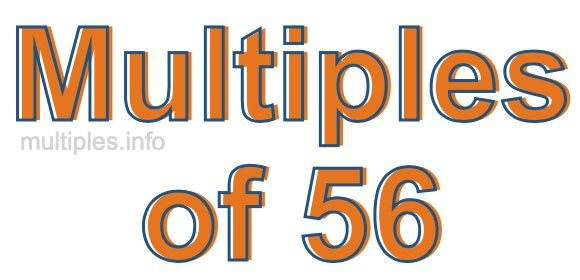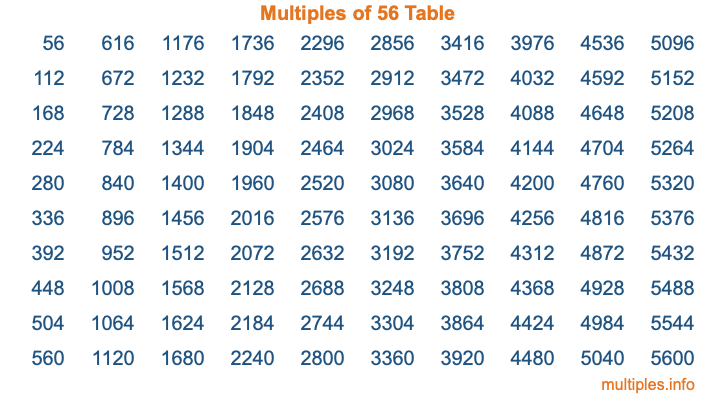Multiples of 56Welcome to the Multiples of 56 page. Here we will first teach you everything you will ever need to know about the multiples of 56, and then give you a study guide summary of everything we taught you to make sure you remember it all. Use this page to look up facts and learn information about the multiples of 56. This page will make you a multiples of fifty-six expert!

Definition of Multiples of 56
Multiples of 56 are all the numbers that when divided by 56 equal an integer. Each of the multiples of 56 are called a multiple. A multiple of 56 is created by multiplying 56 by an integer.

Therefore, to create a list of multiples of 56, you start with 1 multiplied by 56, then 2 multiplied by 56, then 3 multiplied by 56, and so on for as long as you want. Thus, the list of the first five multiples of 56 is 56, 112, 168, 224, and 280. To see a larger list of multiples of 56, see the printable image of Multiples of 56 further down on this page. We also have a category where you can choose any nth multiple of 56.

Multiples of 56 Checker
The Multiples of 56 Checker below checks to see if any number of your choice is a multiple of 56. In other words, it checks to see if there is any number (integer) that when multiplied by 56 will equal your number. To do that, we divide your number by 56. If the the quotient is an integer, then your number is a multiple of 56.

Is  a multiple of 56?

Least Common Multiple of 56 and ...
A Least Common Multiple (LCM) is the lowest multiple that two or more numbers have in common. This is also called the smallest common multiple or lowest common multiple and is useful to know when you are adding our subtracting fractions. Enter one or more numbers below (56 is already entered) to find the LCM.

Check out our LCM Calculator if you need more details about the Least Common Multiple or if you need the LCM for different numbers for adding and subtraction fractions.

nth Multiple of 56
As we stated above, 56 is the first multiple of 56, 112 is the second multiple of 56, 168 is the third multiple of 56, and so on. Enter a number below to find the nth multiple of 56.

th multiple of 56

Multiples of 56 vs Factors of 56
56 is a multiple of 56 and a factor of 56, but that is where the similarities end. All postive multiples of 56 are 56 or greater than 56. All positive factors of 56 are 56 or less than 56.

Below is the beginning list of multiples of 56 and the factors of 56 so you can compare:

Multiples of 56: 56, 112, 168, 224, 280, etc.

Factors of 56: 1, 2, 4, 7, 8, 14, 28, 56

As you can see, the multiples of 56 are all the numbers that you can divide by 56 to get a whole number. The factors of 56, on the other hand, are all the whole numbers that you can multiply by another whole number to get 56.

It's also interesting to note that if a number (x) is a factor of 56, then 56 will also be a multiple of that number (x).

Multiples of 56 vs Divisors of 56
The divisors of 56 are all the integers that 56 can be divided by evenly. Below is a list of the divisors of 56.

Divisors of 56: 1, 2, 4, 7, 8, 14, 28, 56

The interesting thing to note here is that if you take any multiple of 56 and divide it by a divisor of 56, you will see that the quotient is an integer.

Multiples of 56 Table
Below is an image of the first 100 multiples of 56 in a table. The table is in chronological order, column by column. The first column has the first ten multiples of 56, the second column has the next ten multiples of 56, and so on.The Multiples of 56 Table is also referred to as the 56 Times Table or Times Table of 56. You are welcome to print out our table for your studies.

Negative Multiples of 56
Although not often discussed or needed in math, it is worth mentioning that you can make a list of negative multiples of 56 by multiplying 56 by -1, then by -2, then by -3, and so on, to get the following list of negative multiples of 56:

-56, -112, -168, -224, -280, etc.

Multiples of 56 Summary
Below is a summary of important Multiples of 56 facts that we have discussed on this page. To retain the knowledge on this page, we recommend that you read through the summary and explain to yourself or a study partner why they hold true.

There are an infinite number of multiples of 56.

A multiple of 56 divided by 56 will equal a whole number.

56 divided by a factor of 56 equals a divisor of 56.

The nth multiple of 56 is n times 56.

The largest factor of 56 is equal to the first positive multiple of 56.

56 is a multiple of every factor of 56.

56 is a multiple of 56.

A multiple of 56 divided by a divisor of 56 equals an integer.

56 divided by a divisor of 56 equals a factor of 56.

Any integer times 56 will equal a multiple of 56.

Multiples of a Number
Here you can get the multiples of another number, all with the same attention to detail as we did for multiples of 56 on this page.

Multiples of
Multiples of 57
Did you find our page about multiples of fifty-six educational? Do you want more knowledge? Check out the multiples of the next number on our list!